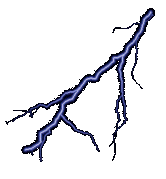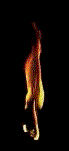# Infinities

### Sep 6th, 2022Infinity is a fun topic to grapple with. We often speak of it in hushed tones or maybe we talk about some of its more interesting characteristics. For instance, infinity + 1 is infinity as is infinity - 1 or even infinity * infinity. The real truth of it though is a lot stranger than that. Helping people see a glimpse of the true nature of sets that grow without boundaries is one of the greatest joys of teaching discrete mathematics and theory of computation. So let’s play with the idea for a second. How can we possibly quantify an infinite set? The answer is that we can explore it all through sets themselves. So what is a set then? A set is just a collection of distinct things. We could have a set of numbers, like the natural numbers: ``````N = { 1, 2, 3, 4, ... } `````` Or we could have a set of just a few things: ``````Digits = { 0, 1, 2, 3, 4, 5, 6, 7, 8, 9 } `````` The number of elements in a set is called its cardinality. For finite sets, this is pretty boring stuff. We just count them. But what about infinite sets? To answer that, let’s think of what happens when sets interact. For example, suppose we had: ``````Q = { 1, 2, 3 } R = { Orange, Banana } `````` We could start paring these things off with each other. In fact, we could get fancy and call this a cartesian product of the sets, but this is just taking the two and making every possible pairing: ``````Q x R = { (1, Orange), (2, Orange), (3, Orange), (1, Banana), (2, Banana), (3, Banana) } `````` Such an object is, of course, itself a set! It’s a set of ordered pairs `(q, r)` where `q` is in `Q` and `r` is in `R`. When we have something that establishes ordered pairs between two sets, we call it a correspondence. Oh, but some correspondences are special! If we constrain it so that each `q` only appears only appears in at most one ordered pair, we call this correspondance a function! So `Q x R` is not a function, but we could make some functions from its subsets: ``````F1: Q -> R = { (1, Orange), (2, Banana), (3, Banana) } F2: Q -> R = { (1, Orange), (2, Banana), (3, Orange) } `````` Get the idea? Good! Now here’s where it gets interesting. We can classify functions into three basic categories: injective, surjective, and bijective. They sound horrible, but really they are very simple. An injective function `f: A -> B` is a function where the mappings in `B` are unique. That is, if `f(x) = f(y)` then it must be true that `x = y`. Take for instance the following: ``````A ---> Exceptional B ---> Acceptable C ---> Meh `````` This is a function which is injective. Each element in B is only covered once. Now what about our sets `Q` and `R`? Neither `F1` nor `F2` are injective. In fact, we can’t make an injective function with these sets! Why not? Well, there aren’t enough `R`s to go around! If we try to make an injective function we run into a problem: ``````1 --> Orange 2 --> Banana 3 `````` or ``````1 2 --> Orange 3 --> Banana `````` and so on. We just can’t build it! So that means that injections are only possible if there are at least as many elements in the right hand side as there are in the left hand side. Or, we could get really formal and state: ``````An injective function f: A -> B exists if and only if |A| <= |B| `````` (Those “`|`” symbols just mean the number of elements in the set.) Ok, so that’s injection. Surjection is the other side of things. It says that for every element in right hand side, there is one matching element in the left hand side. So in our `Q` and `R` examples, `F1` and `F2` are both surjections. It should be easy to see that surjection requires that there enough on the left to go around. So: ``````A 90 B 80 C 70 D `````` Has some surjections and: ``````A Aardvark B Bat Cat `````` does not. So then we can say: ``````A surjective function f: A -> B exists if and only if |A| >= |B| `````` A bijective function is a function that is both injective and surjective. Applying what we have learned above, we can easily see that: ``````A bijective function f: A -> B exists if and only if |A| == |B| `````` (This is because `f` would be both injective and surjective, thus `|A| <= |B|` and `|A| >= |B|`, which is only possible if `|A| == |B|`) Now we have some powerful tools! Let’s play with them on infinite sets. Suppose we say that `E` is the set of all even natural numbers: ``````E = { x in N : x is divisble by 2 } `````` Now, we know that only half of all of the numbers in `N` are even, but there is an infinite supply of them. So how do the sets stack up? Well, let’s ask ourselves “can we establish a bijection between the sets?” It turns out we can! ``````f(n) = 2n `````` This function will always give us an even number because it is always multiplying things by 2. And it gets all of the even numbers: ``````f(1) = 2 f(2) = 4 f(3) = 6 `````` We can easily see that because any number in between would have to be odd because they would be of the form `2n+1`, so it must be the case that this is a bijection. We can work it backwards and forwards, and so we find that: ``````|E| = |N| `````` All is right with the world. Infinity is Infinity, but is it always so? What about real numbers? Those wacky decimals that we can write: 100.5, 3.14159, 1.412789, … and so on. Let’s focus in on the real numbers between 0 and 1. These can all be written like this: ``````0.d1 d2 d3 d4 d5 ... `````` where each `di` is a digit from `{0, 1, 2, 3, 4, 5, 6, 7, 8, 9}`. In fact, we can say that all real numbers can be written by an infiite chain of numbers which either go on forever (like 1/3) or have infinite zeros after their supposedly “terminating” digit. So really, every real number is an infinite sequence of digits! ``````d1 d2 d3 d4 ... di ... `````` The real numbers themselves are clearly infinite, but are the same sort of infinite as the natural numbers? If they were, we could establish some function: ``````f(1) = R1 f(2) = R2 f(3) = R3 f(4) = R4 ... `````` So can we build it? If we could, it would have to be the case that we could build out a grid of digits: ``````f(1) = r1,1 r1,2 r1,3 ... r1,i ... f(2) = r2,1 r2,2 r2,3 ... r2,i ... f(3) = r3,1 r3,2 r3,3 ... r3,i ... ... f(j) = rj,1 rj,2 rj,3 ... 4j,i ... `````` But here’s the deal. A guy named Georg Cantor realized that we can’t really build this function! Why not? Becuase no matter how we build, we can construct a number that’s not in the list! Here’s how we do it: ``````B = b1 b2 b3 b4 b5 ... bi ... `````` where for every: ``````bi = some digit other than what is at ri,i `````` So b1 = some digit other than r1,1 b2 = some digit other than r2,2 and so on. Thus `f` cannot possibly generate `B` because it differs in every digit from all the other numbers in the set. (This is called the diagonal argument because we alter the numbers along this infinitely long diagonal.) Ok, so we know that there is no bijection. So is there a surjection? No, because `B` gets missed every time we try to build `f`. All the natural numbers are mapped though, so we have an injective function! So that means that: |N| <= |R| and we know that |N| =/= |R| so it must be the case that: |N| < |R| That is to say, the infinite set N is smaller than the infinite set R! Ack! Infinites come in different sizes. We have the countable infinite sets, those that are the same size as the set of natural numbers and then we have those infinite sets that are bigger than the set of natural numbers. We call the bigger ones “Uncountably Infinite.” (How’s that for a badass title?) The mathematicians of the 1870s did not respond well to this. In fact, by the turn of the 20th century they were in full-on crisis mode. Some were trying to preseve Cantor, some were trying to redefine math in a way that would make Cantor wrong. As for Cantor himself, he died in poverty in 1918. `*sigh*` I suppose a prophet really is never appreciated in their own time. So there it is. Infinites are not all equal, some are bigger than others. I hope you enjoyed my slightly informal tour of the thing that confounded a a lot of great minds over 100 years ago. This, in turn, gave rise to computer science. How, you ask? Well that’s a story for another time.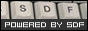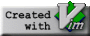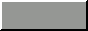Visit me on Mastodon.